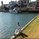1558 views
Ultimate Oscillator with bands present at the 30, 50, and 70 pt levels.
Personally use this every time, created a script to hard code these lines so I wouldn't need to redraw them all the time.
Enjoy
Open-source script

In true TradingView spirit, the author of this script has published it open-source, so traders can understand and verify it. Cheers to the author! You may use it for free, but reuse of this code in a publication is governed by House Rules. You can favorite it to use it on a chart.

Want to use this script on a chart?
study(title="Ultimate Oscillator", shorttitle="UO")
length7 = input(7, minval=1), length14 = input(14, minval=1), length28 = input(28, minval=1)
average(bp, tr_, length) => sum(bp, length) / sum(tr_, length)
high_ = max(high, close)
low_ = min(low, close)
bp = close - low_
tr_ = high_ - low_
avg7 = average(bp, tr_, length7)
avg14 = average(bp, tr_, length14)
avg28 = average(bp, tr_, length28)
out = 100 * (4*avg7 + 2*avg14 + avg28)/7
plot(out, color=red, title="UO")
band1 = hline(70)
band2 = hline(50,color=yellow)
band0 = hline(30)
fill(band1, band0, color=orange, transp=90)